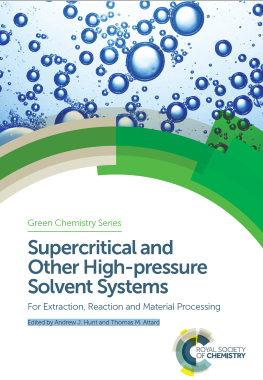## Simple scCO2 Entrainer Calculations

### Quick Start

It turns out to be rather simple to analyse entrainer effects in scCO2 via a straightforward set of calculations. The background and full theory are described in the chapter by Abbott and Shimizu, "Understanding entrainer effects in scCO2", in Supercritical and Other High-pressure Solvent Systems, For Extraction, Reaction and Material Processing, Edited by Andrew J. Hunt and Thomas M. Attard, Royal Society of Chemistry Green Chemistry Series, August 2018.

### Simple scCO2 Entrainer Calculations

P bar
T °K
y2%
xu0
f = xue/xu0
P MPa
T °C
ρCO2 S&W g/cc
xue
kav
Gu2approx cc/mole
K
A
B
k
m
Tcalc

The scCO2 app provides a full analysis of detailed entrainer effect datasets in order to derive as many parameters as possible from the data. Here we are using the bare minimum of data and some simplifying assumptions which turn out to be surprisingly good at providing key insights into scCO2 entrainers.

The starting point is the Chrastil formula for the basic solubility, S, of a solute in scCO2. It depends on the density, ρ, of the scCO2, the temperature, T and three parameters, K, A and B via.

S=ρKexp(A/T+B)

To add the entrainer effect, we use a linearised Sovová equation which loses some accuracy against the generalised version but which gains in universality so we can compare like with like. We use a constant, kav and link to a commonly given factor f which is the ratio of the solubility with the entrainer is Se to that without entrainer, so f=Se/S, at a given mole fraction of entrainer, y2

Se=S(1+kavy2)

### The key to understanding entrainers

As the book has not yet been published, it's not appropriate to discuss the significance of using kav. For the moment, note that it allows us to provide an objective measure of the efficacy of entrainers, unlike the f values which are concentration specific. From an anlysis of kav values it is possible to create a unified explanation of entrainer effects and this will be discussed here once the book is published.

### An app in two parts

The two rows above the graphs do the required analysis of the simplified entrainer calculation. You enter the experimental conditions (P, T and mole % entrainer y2, the experimental solubility with no entrainer, xu0 and from the standard Span & Wagner EoS you get the density, from your f value you get the solubility in the entrainer, xue and the kav value for the modified Sovová equation. Finally, to fit in with the KB scCO2 analysis you get an estimate of the KBI, Gu2

Below the graphs you get the chance to implement a full Chrastil and Sovová simulation so you can compare the approximate value with any experimental data you have available. This is a rather powerful modelling tool of general interest.

To link the two parts, you can enter the kav from the data above and a value of m=1, allowing you to compare a real Sovová simulation with your simulated results. The standard, and much-used Sovová equation is:

Se=S+k.y2m.Sn

Experimentally, n is somewhere between 0.5 and 1 but for simplicity, the app assumes that n=1.